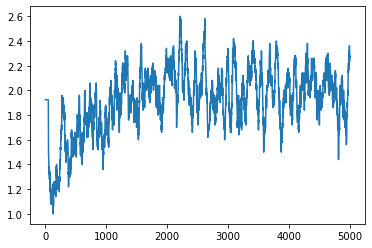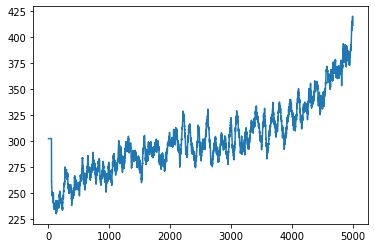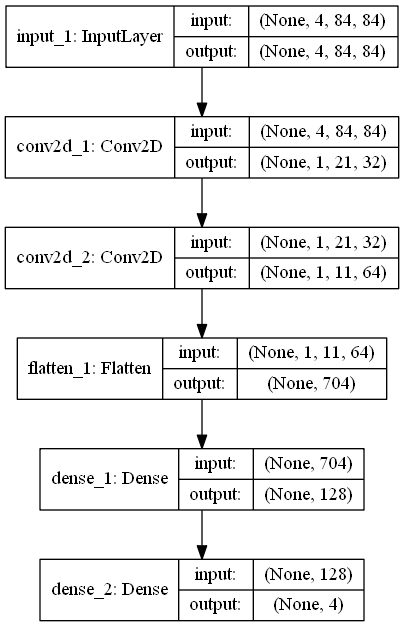# Introduction

I will try to break the block of OpenAI Gym.Image borrowed from OpenAI https://gym.openai.com/videos/2019-10-21--mqt8Qj1mwo/Breakout-v0/poster.jpg

This time we will use Keras to build and train an Actor-Critic model. Keras is a good sample for beginners as it is an API that makes it easier to introduce deep learning, and there are few variables and functions that you need to adjust yourself.

The Actor-Critic model is one of the reinforcement learning models. A detailed explanation is posted on the Tensorflow blog. (https://www.tensorflow.org/tutorials/reinforcement_learning/actor_critic) However, unlike the CartPole example of the link, we will break the block this time. I use a CNN network because there are far more breakouts (84x84 images per frame) than four variables like CartPole. The detailed configuration will be explained later.

# result

I will post the result first. 1 training = 1 game, 5000 trainings over 8 hours (episode). The result takes the average of the last 50 times. The first sheet is the score, and the second sheet is the number of steps (time step).The score seems to have increased a little, but the number of steps gave a considerably better result. By the way, at first, I can't get an average of 50 times, so it looks abnormal.

# manner

Gameplay and reinforcement learning will be explained separately. The gameplay part plays the game in the Gym environment of OpenAI. The reinforcement learning part trains the variables received from the game and reflects the predicted actions in the game.

## Gameplay

``````import gym
import random
import numpy as np

env = gym.make('Breakout-v4')
episode_limit = 5000
random_step = 20
timestep_limit = 100000 #Restricted to not play forever
model_train = 1 #No training when set to 0(Just random play)
log_save = 1 #If set to 0, the log will not be saved.
log_path = 'atari_ac_log.txt'
score_list = []
step_list = []

for episode in range(episode_limit):
#Reset the environment before playing every time
observation = env.reset()
score = 0

#Do nothing with the first random number of steps to randomize the position of the ball
for _ in range(random.randint(1, random_step)):
observation_last = observation
observation, _, _, _ = env.step(0)
#Encode the observed data of the action(I will explain later)
state = encode_initialize(observation, observation_last)

for timestep in range(timestep_limit):
observation_last = observation
#Get the action to predict from the model(I will explain later)
action = action_choose(state[np.newaxis, :], epsilon, episode, action_space)
#Act based on predicted actions
observation, reward, done, _ = env.step(action)
#Encode the observed data of the action(I will explain later)
state_next = encode(observation, observation_last, state)
if model_train == 1:
#Send the observation data of the behavior to the model for learning(I will explain later)
network_learn(state[np.newaxis, :], action, reward, state_next[np.newaxis, :], done)
state = state_next

score += reward
#End of game or time step_Reach limit(forced termination)
if done or timestep == timestep_limit - 1:
#Record results
score_list.append(score)
step_list.append(timestep)
if log_save == 1:
log(log_path, episode, timestep, score)
print('Episode {} Timestep {} Score {}'.format(episode + 1, timestep, score))
break

#A function that randomizes actions to a certain degree(I will explain later)
epsilon = epsilon_reduce(epsilon, episode)

env.close()
``````

## Reinforcement learning

The encoded part is grayscale converted, resized and composited with 4 consecutive 84x84 images (frames). This means that you can record the action of the ball more and it is easier to train.

``````from skimage.color import rgb2gray
from skimage.transform import resize

frame_length = 4
frame_width = 84
frame_height = 84

def encode_initialize(observation, last_observation):
processed_observation = np.maximum(observation, last_observation)
processed_observation_resize = np.uint8(resize(rgb2gray(processed_observation), (frame_width, frame_height)) * 255)
state = [processed_observation_resize for _ in range(frame_length)]
state_encode = np.stack(state, axis=0)
return state_encode

def encode(observation, last_observation, state):
processed_observation = np.maximum(observation, last_observation)
processed_observation_resize = np.uint8(resize(rgb2gray(processed_observation), (frame_width, frame_height)) * 255)
state_next_return =  np.reshape(processed_observation_resize, (1, frame_width, frame_height))
state_encode = np.append(state[1:, :, :], state_next_return, axis=0)
return state_encode
``````

The network and training part is the most difficult, but it looks like this when first represented in a diagram.The pre-encoded data is sent to the Conv2D layer of 2 layers. Then flatten it and send it to the two Dense layers. Finally, there are four outputs (NOPE, FIRE, LEFT, RIGHT according to the OpenAI Gym Breakout-v4 specifications). By the way, the activation function is relu, the loss function is as per the paper, and the learning rate is 0.001 for both actor and critic.

``````from keras import backend as K
from keras.layers import Dense, Input, Flatten, Conv2D
from keras.models import Model, load_model
from keras.optimizers import Adam
from keras.utils import plot_model

verbose = 0
action_dim = env.action_space.n
action_space = [i for i in range(action_dim)] # ['NOOP', 'FIRE', 'RIGHT', 'LEFT']
discount = 0.97
actor_lr = 0.001 #Actor learning rate
critic_lr = 0.001 #critic learning rate
pretrain_use = 0 #Set to 1 to use the trained model
actor_h5_path = 'atari_ac_actor.h5'
critic_h5_path = 'atari_ac_critic.h5'

#Model building
input = Input(shape=(frame_length, frame_width, frame_height))
delta = Input(shape=)
con1 = Conv2D(32, (8, 8), strides=(4, 4), padding='same', activation='relu')(input)
con2 = Conv2D(64, (4, 4), strides=(2, 2), padding='same', activation='relu')(con1)
fla1 = Flatten()(con2)
dense = Dense(128, activation='relu')(fla1) #prob,Share value
prob = Dense(action_dim, activation='softmax')(dense) #actor part
value = Dense(1, activation='linear')(dense) #critic part

#Definition of loss function
def custom_loss(y_true, y_pred):
out = K.clip(y_pred, 1e-8, 1-1e-8) #Set limits
log_lik = y_true * K.log(out) #Policy gradient
return K.sum(-log_lik * delta)

if pretrain_use == 1:
#Use a trained model
actor = load_model(actor_h5_path, custom_objects={'custom_loss': custom_loss}, compile=False)

actor = Model(inputs=[input, delta], outputs=[prob])
critic = Model(inputs=[input], outputs=[value])
policy = Model(inputs=[input], outputs=[prob])

#Predict action
def action_choose(state, epsilon, episode, action_space):
#epsilon first set to 1 and gradually decrease
#Compared to random numbers every time you act
#Take random action if epsilon is larger
if epsilon >= random.random() or episode < initial_replay:
action = random.randrange(action_dim)
else:
probabiliy = policy.predict(state)
#Predicted results have probabilities for each of the four actions
#Choose an action according to that probability
action = np.random.choice(action_space, p=probabiliy)
return action

#Learn data
def network_learn(state, action, reward, state_next, done):
reward_clip = np.sign(reward)
critic_value = critic.predict(state)
critic_value_next = critic.predict(state_next)

target = reward_clip + discount * critic_value_next * (1 - int(done))
delta =  target - critic_value
actions = np.zeros([1, action_dim])
actions[np.arange(1), action] = 1

actor.fit([state, delta], actions, verbose=verbose)
critic.fit(state, target, verbose=verbose)
``````

This part is not directly related to reinforcement learning as another function, but I will write it together.

``````import matplotlib.pyplot as plt

model_save = 1 #0 does not save the model
score_avg_freq = 50
epsilon_start = 1.0 #Probability of starting epsilon
epsilon_end = 0.1 #epsilon lowest probability(At least 10%Random action with)
epsilon_step = episode_limit
epsilon = 1.0
epsilon_reduce_step = (epsilon_start - epsilon_end) / epsilon_step
initial_replay = 200
actor_graph_path = 'atari_ac_actor.png'
critic_graph_path = 'atari_ac_critic.png'
policy_graph_path = 'atari_ac_policy.png'

#epsilon lowering function
def epsilon_reduce(epsilon, episode):
if epsilon > epsilon_end and episode >= initial_replay:
epsilon -= epsilon_reduce_step
return epsilon

#Write a log
def log(log_path, episode, timestep, score):
logger = open(log_path, 'a')
if episode == 0:
logger.write('Episode Timestep Score\n')
logger.write('{} {} {}\n'.format(episode + 1, timestep, score))
logger.close()

if pretrain_use == 1:
if model_save == 1:
actor.save(actor_h5_path)
critic.save(critic_h5_path)
else:
if model_save == 1:
actor.save(actor_h5_path)
critic.save(critic_h5_path)
#Output model configuration to diagram
plot_model(actor, show_shapes=True, to_file=actor_graph_path)
plot_model(critic, show_shapes=True, to_file=critic_graph_path)
plot_model(policy, show_shapes=True, to_file=policy_graph_path)

#Output the result to the figure
xaxis = []
score_avg_list = []
step_avg_list = []
for i in range(1, episode_limit + 1):
xaxis.append(i)
if i < score_avg_freq:
score_avg_list.append(np.mean(score_list[:]))
step_avg_list.append(np.mean(step_list[:]))
else:
score_avg_list.append(np.mean(score_list[i - score_avg_freq:i]))
step_avg_list.append(np.mean(step_list[i - score_avg_freq:i]))

plt.plot(xaxis, score_avg_list)
plt.show()

plt.plot(xaxis, step_avg_list)
plt.show()
``````